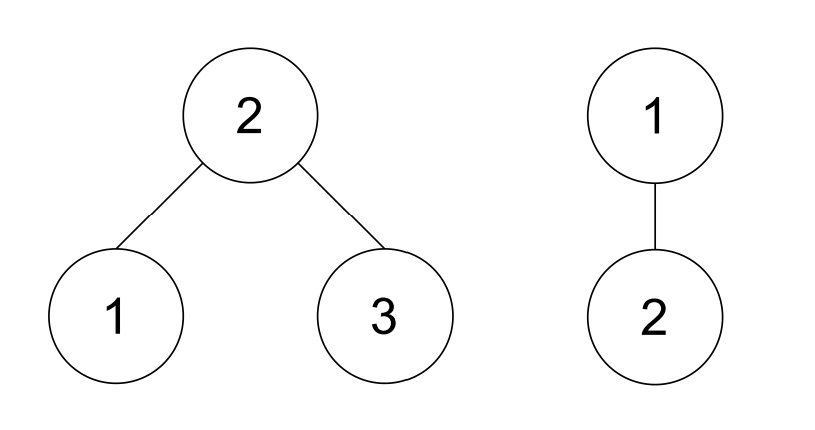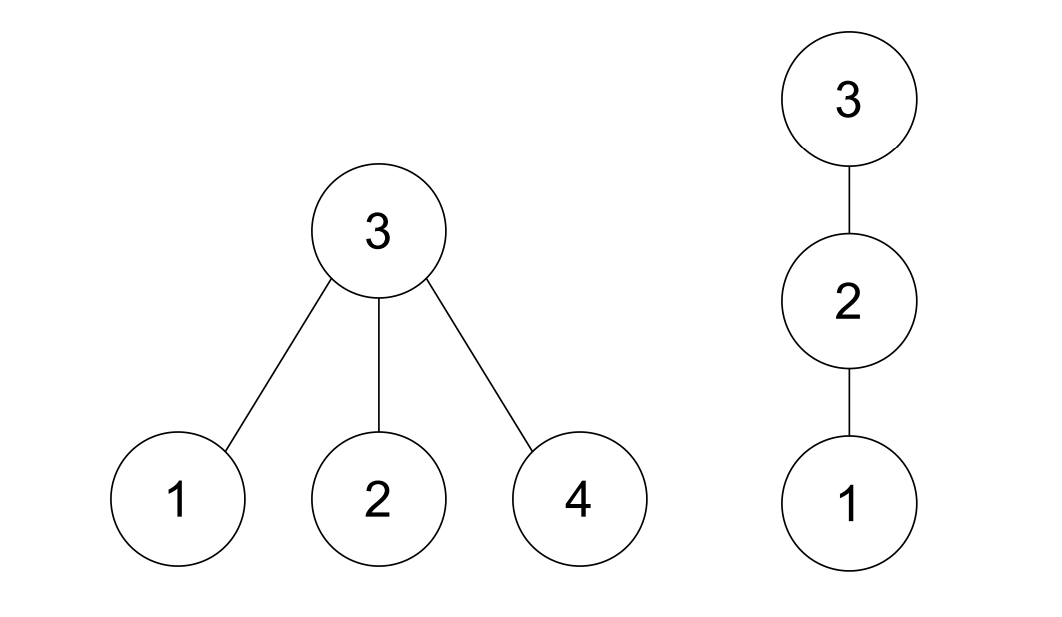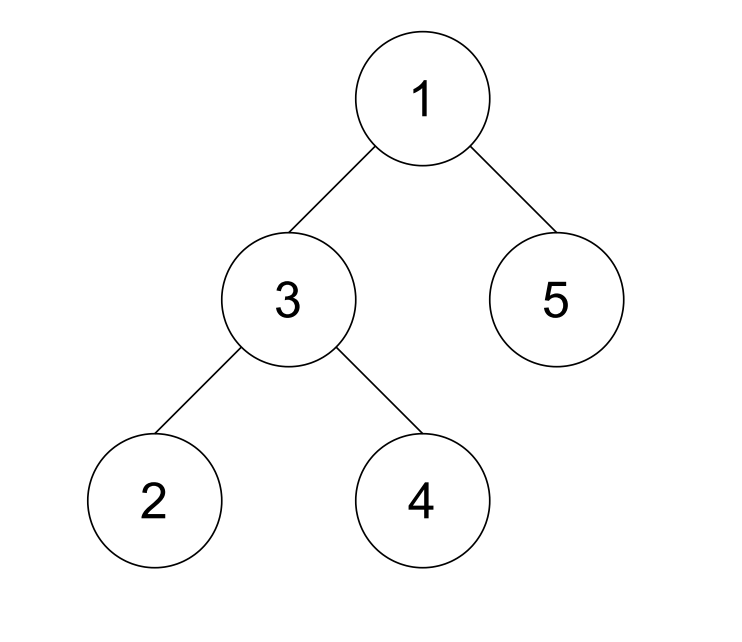# #767. 【NOI2022】挑战 NPC Ⅱ

• $G'$ 连通。
• $G'$ 包含 $G$ 中的根节点（也就是说 $G$ 根节点在删除过程中没有被删除）。
• $G'$ 和 $H$ 同构（也就是说存在一种让 $G'$ 中点重标号的方式，使得重标号得到的图和 $H$ 完全相同，且 $G$ 中的根节点经过重标号后恰好为 $H$ 的根节点）。

### 样例一

#### input

0 3 1
3
2 -1 2
2
-1 1
4
3 3 -1 3
3
2 3 -1
5
-1 1 5 5 1
5
2 3 -1 3 2

#### output

Yes
No
Yes

#### explanation### 子任务

$n_1, n_2$ $\sum n_1$ 测试点 $k$ 特殊性质
$\le 8$ $\le 500$ $1,2,3$ $\le 0$
$4,5,6$ $\le 5$
$\le 16$ $\le 10^3$ $7,8$ $\le 0$
$9,10$ $\le 5$
$\le 150$ $\le 10^4$ $11$ $\le 0$
$12$ $\le 1$
$13$ $\le 5$
$\le 10^5$ $\le 5 \times 10^5$ $14,15,16$ $\le 0$ A
$17,18,19,20$ B
$21$ $\le 1$
$22,23$ $\le 3$
$24, 25$ $\le 5$

• 特殊性质 A：保证有根树 $G$ 每个节点要么是叶节点，要么有恰好 $1$ 个儿子结点；另一种等价的表述是有根树 $G$ 构成了一条链，且根节点为链的一个端点。
• 特殊性质 B：保证有根树 $G$ 每个节点要么是叶节点，要么有恰好 $2$ 个儿子结点，同时保证 $G$ 的每一个叶节点深度均相同；另一种等价的表述是有根树 $G$ 构成一棵完全二叉树，且根节点为完全二叉树的根节点。